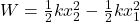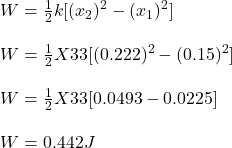## A spring with spring constant of 33 N/m is stretched 0.15 m from its equilibrium position. How much work must be done to stretch it an addit

Question

A spring with spring constant of 33 N/m is stretched 0.15 m from its equilibrium position. How much work must be done to stretch it an additional 0.072 m? 1. 0.420 J 2. 0.486 J 3. 0.552 J 4. 0.398 J 5. 0.376 J 6. 0.464 J 7. 0.574 J

in progress 0
2 months 2021-07-30T22:07:48+00:00 1 Answers 1 views 0

1. Work done is 0.442J

Explanation:

Given:

Spring constant, k = 33 N/m

Distance, x₁ = 0.15m

Additional distance, x₂ = 0.072 m

Total distance = 0.15 + 0.072 m

= 0.222 m

Work done, W = ?

We can calculate work done by the formulaOn substituting the value we get:Therefore, work done is 0.442J